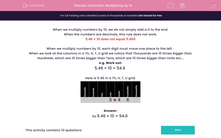# Decimals: Multiplying by 10

In this worksheet, students multiply numbers by 10 by shifting digits one place to the left in a grid.Key stage:  KS 2

Curriculum topic:   Maths and Numerical Reasoning

Curriculum subtopic:   Decimals

Difficulty level:#### Worksheet Overview

When we multiply numbers by 10, we do not simply add a 0 to the end.

When the numbers are decimals, this rule does not work.

5.46 × 10 does not equal 5.460

When we multiply numbers by 10, each digit must move one place to the left.

When we look at the columns in a Th, H, T, U grid we notice that Thousands are 10 times bigger than Hundreds, which are 10 times bigger than Tens, which are 10 times bigger than Units etc...

e.g. Work out:

5.46 × 10 = 54.6

Here is 5.46 in a Th, H, T, U grid.